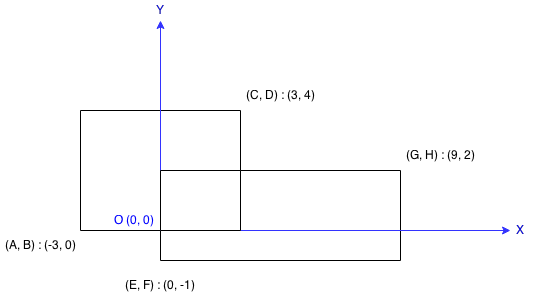# Rectangle Area

### 描述​

Find the total area covered by two rectilinear rectangles in a 2D plane.

Each rectangle is defined by its bottom left corner and top right corner as shown in the figure.Assume that the total area is never beyond the maximum possible value of int.

### 代码​

// Rectangle Area// Time Complexity: O(1), Space Complexity: O(1)public class Solution {    public int computeArea(int A, int B, int C, int D, int E, int F, int G, int H) {        final int area = (C - A) * (D- B) + (G - E) * (H - F);        // prevent overflow        if (C < E || G < A || D < F || H < B) return area;        final int intersection = Math.max(Math.min(C, G) - Math.max(A, E), 0) *                Math.max(Math.min(D, H) - Math.max(B, F), 0);        return area - intersection;    }}